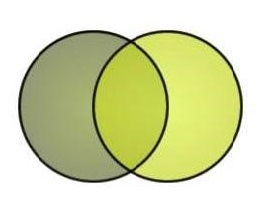mathematical proof

n=2

a: mathematical proof ~
b: a piece of music

What:

"A mathematical proof is like a piece of music: there is really no substitute for seeing it develop in real time."

Useful?
Writer: Not Stated
LCC:
Where:
Date: Oct 14 2015 6:40 PM

a: mathematical proof ~
b: program to be run on a biological computer

What:

Useful?
Writer: Not Stated
LCC: